# Phase-shift keying

Phase-shift keying
Passband modulation v · d · e
Analog modulation
AM · SSB · QAM · FM · PM · SM
Digital modulation
FSK · MFSK · ASK · OOK · PSK · QAM
MSK · CPM · PPM · TCM · SC-FDE
CSS · DSSS · FHSS · THSS
line coding, PAM, PWM, PCM

Phase-shift keying (PSK) is a digital modulation scheme that conveys data by changing, or modulating, the phase of a reference signal (the carrier wave).

Any digital modulation scheme uses a finite number of distinct signals to represent digital data. PSK uses a finite number of phases, each assigned a unique pattern of binary digits. Usually, each phase encodes an equal number of bits. Each pattern of bits forms the symbol that is represented by the particular phase. The demodulator, which is designed specifically for the symbol-set used by the modulator, determines the phase of the received signal and maps it back to the symbol it represents, thus recovering the original data. This requires the receiver to be able to compare the phase of the received signal to a reference signal — such a system is termed coherent (and referred to as CPSK).

Alternatively, instead of using the bit patterns to set the phase of the wave, it can instead be used to change it by a specified amount. The demodulator then determines the changes in the phase of the received signal rather than the phase itself. Since this scheme depends on the difference between successive phases, it is termed differential phase-shift keying (DPSK). DPSK can be significantly simpler to implement than ordinary PSK since there is no need for the demodulator to have a copy of the reference signal to determine the exact phase of the received signal (it is a non-coherent scheme). In exchange, it produces more erroneous demodulations. The exact requirements of the particular scenario under consideration determine which scheme is used.

## Introduction

There are three major classes of digital modulation techniques used for transmission of digitally represented data:

All convey data by changing some aspect of a base signal, the carrier wave (usually a sinusoid), in response to a data signal. In the case of PSK, the phase is changed to represent the data signal. There are two fundamental ways of utilizing the phase of a signal in this way:

• By viewing the phase itself as conveying the information, in which case the demodulator must have a reference signal to compare the received signal's phase against; or
• By viewing the change in the phase as conveying information — differential schemes, some of which do not need a reference carrier (to a certain extent).

A convenient way to represent PSK schemes is on a constellation diagram. This shows the points in the complex plane where, in this context, the real and imaginary axes are termed the in-phase and quadrature axes respectively due to their 90° separation. Such a representation on perpendicular axes lends itself to straightforward implementation. The amplitude of each point along the in-phase axis is used to modulate a cosine (or sine) wave and the amplitude along the quadrature axis to modulate a sine (or cosine) wave.

In PSK, the constellation points chosen are usually positioned with uniform angular spacing around a circle. This gives maximum phase-separation between adjacent points and thus the best immunity to corruption. They are positioned on a circle so that they can all be transmitted with the same energy. In this way, the moduli of the complex numbers they represent will be the same and thus so will the amplitudes needed for the cosine and sine waves. Two common examples are "binary phase-shift keying" (BPSK) which uses two phases, and "quadrature phase-shift keying" (QPSK) which uses four phases, although any number of phases may be used. Since the data to be conveyed are usually binary, the PSK scheme is usually designed with the number of constellation points being a power of 2.

### Definitions

For determining error-rates mathematically, some definitions will be needed:

Q(x) will give the probability that a single sample taken from a random process with zero-mean and unit-variance Gaussian probability density function will be greater or equal to x. It is a scaled form of the complementary Gaussian error function:$Q(x) = \frac{1}{\sqrt{2\pi}}\int_{x}^{\infty}e^{-t^{2}/2}dt = \frac{1}{2}\,\operatorname{erfc}\left(\frac{x}{\sqrt{2}}\right),\ x\geq{}0$.

The error-rates quoted here are those in additive white Gaussian noise (AWGN). These error rates are lower than those computed in fading channels, hence, are a good theoretical benchmark to compare with.

## Applications

Owing to PSK's simplicity, particularly when compared with its competitor quadrature amplitude modulation, it is widely used in existing technologies.

The wireless LAN standard, IEEE 802.11b-1999, uses a variety of different PSKs depending on the data-rate required. At the basic-rate of 1 Mbit/s, it uses DBPSK (differential BPSK). To provide the extended-rate of 2 Mbit/s, DQPSK is used. In reaching 5.5 Mbit/s and the full-rate of 11 Mbit/s, QPSK is employed, but has to be coupled with complementary code keying. The higher-speed wireless LAN standard, IEEE 802.11g-2003 has eight data rates: 6, 9, 12, 18, 24, 36, 48 and 54 Mbit/s. The 6 and 9 Mbit/s modes use OFDM modulation where each sub-carrier is BPSK modulated. The 12 and 18 Mbit/s modes use OFDM with QPSK. The fastest four modes use OFDM with forms of quadrature amplitude modulation.

Because of its simplicity BPSK is appropriate for low-cost passive transmitters, and is used in RFID standards such as ISO/IEC 14443 which has been adopted for biometric passports, credit cards such as American Express's ExpressPay, and many other applications.

Bluetooth 2 will use π / 4-DQPSK at its lower rate (2 Mbit/s) and 8-DPSK at its higher rate (3 Mbit/s) when the link between the two devices is sufficiently robust. Bluetooth 1 modulates with Gaussian minimum-shift keying, a binary scheme, so either modulation choice in version 2 will yield a higher data-rate. A similar technology, IEEE 802.15.4 (the wireless standard used by ZigBee) also relies on PSK. IEEE 802.15.4 allows the use of two frequency bands: 868–915 MHz using BPSK and at 2.4 GHz using OQPSK.

Notably absent from these various schemes is 8-PSK. This is because its error-rate performance is close to that of 16-QAM — it is only about 0.5 dB better[citation needed] — but its data rate is only three-quarters that of 16-QAM. Thus 8-PSK is often omitted from standards and, as seen above, schemes tend to 'jump' from QPSK to 16-QAM (8-QAM is possible but difficult to implement).

Included among the exceptions is Hughnet satellite ISP. For example, the model HN7000S modem (on KU-band satcom) uses 8-PSK modulation.

## Binary phase-shift keying (BPSK)Constellation diagram example for BPSK.

BPSK (also sometimes called PRK, Phase Reversal Keying, or 2PSK) is the simplest form of phase shift keying (PSK). It uses two phases which are separated by 180° and so can also be termed 2-PSK. It does not particularly matter exactly where the constellation points are positioned, and in this figure they are shown on the real axis, at 0° and 180°. This modulation is the most robust of all the PSKs since it takes the highest level of noise or distortion to make the demodulator reach an incorrect decision. It is, however, only able to modulate at 1 bit/symbol (as seen in the figure) and so is unsuitable for high data-rate applications when bandwidth is limited.

In the presence of an arbitrary phase-shift introduced by the communications channel, the demodulator is unable to tell which constellation point is which. As a result, the data is often differentially encoded prior to modulation.

### Implementation

The general form for BPSK follows the equation:$s_n(t) = \sqrt{\frac{2E_b}{T_b}} \cos(2 \pi f_c t + \pi(1-n )), n = 0,1.$

This yields two phases, 0 and π. In the specific form, binary data is often conveyed with the following signals:$s_0(t) = \sqrt{\frac{2E_b}{T_b}} \cos(2 \pi f_c t + \pi ) = - \sqrt{\frac{2E_b}{T_b}} \cos(2 \pi f_c t)$ for binary "0"$s_1(t) = \sqrt{\frac{2E_b}{T_b}} \cos(2 \pi f_c t)$ for binary "1"

where fc is the frequency of the carrier-wave.

Hence, the signal-space can be represented by the single basis function$\phi(t) = \sqrt{\frac{2}{T_b}} \cos(2 \pi f_c t)$

where 1 is represented by$\sqrt{E_b} \phi(t)$ and 0 is represented by$-\sqrt{E_b} \phi(t)$. This assignment is, of course, arbitrary.

This use of this basis function is shown at the end of the next section in a signal timing diagram. The topmost signal is a BPSK-modulated cosine wave that the BPSK modulator would produce. The bit-stream that causes this output is shown above the signal (the other parts of this figure are relevant only to QPSK).

### Bit error rate

The bit error rate (BER) of BPSK in AWGN can be calculated as:$P_b = Q\left(\sqrt{\frac{2E_b}{N_0}}\right)$ or$P_b = \frac{1}{2} \operatorname{erfc} \left( \sqrt{\frac{E_b}{N_0}}\right)$

Since there is only one bit per symbol, this is also the symbol error rate.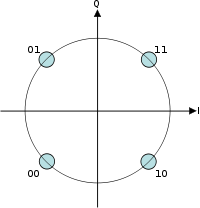Constellation diagram for QPSK with Gray coding. Each adjacent symbol only differs by one bit.

Sometimes this is known as quaternary PSK, quadriphase PSK, 4-PSK, or 4-QAM. (Although the root concepts of QPSK and 4-QAM are different, the resulting modulated radio waves are exactly the same.) QPSK uses four points on the constellation diagram, equispaced around a circle. With four phases, QPSK can encode two bits per symbol, shown in the diagram with gray coding to minimize the bit error rate (BER) — sometimes misperceived as twice the BER of BPSK.

The mathematical analysis shows that QPSK can be used either to double the data rate compared with a BPSK system while maintaining the same bandwidth of the signal, or to maintain the data-rate of BPSK but halving the bandwidth needed. In this latter case, the BER of QPSK is exactly the same as the BER of BPSK - and deciding differently is a common confusion when considering or describing QPSK.

Given that radio communication channels are allocated by agencies such as the Federal Communication Commission giving a prescribed (maximum) bandwidth, the advantage of QPSK over BPSK becomes evident: QPSK transmits twice the data rate in a given bandwidth compared to BPSK - at the same BER. The engineering penalty that is paid is that QPSK transmitters and receivers are more complicated than the ones for BPSK. However, with modern electronics technology, the penalty in cost is very moderate.

As with BPSK, there are phase ambiguity problems at the receiving end, and differentially encoded QPSK is often used in practice.

### Implementation

The implementation of QPSK is more general than that of BPSK and also indicates the implementation of higher-order PSK. Writing the symbols in the constellation diagram in terms of the sine and cosine waves used to transmit them:$s_n(t) = \sqrt{\frac{2E_s}{T_s}} \cos \left ( 2 \pi f_c t + (2n -1) \frac{\pi}{4}\right ),\quad n = 1, 2, 3, 4.$

This yields the four phases π/4, 3π/4, 5π/4 and 7π/4 as needed.

This results in a two-dimensional signal space with unit basis functions$\phi_1(t) = \sqrt{\frac{2}{T_s}} \cos (2 \pi f_c t)$$\phi_2(t) = \sqrt{\frac{2}{T_s}} \sin (2 \pi f_c t)$

The first basis function is used as the in-phase component of the signal and the second as the quadrature component of the signal.

Hence, the signal constellation consists of the signal-space 4 points$\left ( \pm \sqrt{E_s/2}, \pm \sqrt{E_s/2} \right ).$

The factors of 1/2 indicate that the total power is split equally between the two carriers.

Comparing these basis functions with that for BPSK shows clearly how QPSK can be viewed as two independent BPSK signals. Note that the signal-space points for BPSK do not need to split the symbol (bit) energy over the two carriers in the scheme shown in the BPSK constellation diagram.

QPSK systems can be implemented in a number of ways. An illustration of the major components of the transmitter and receiver structure are shown below.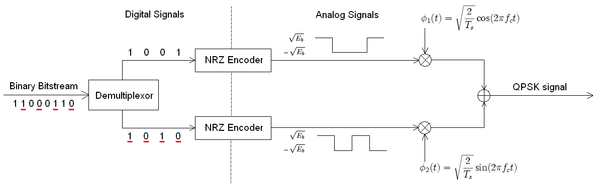Conceptual transmitter structure for QPSK. The binary data stream is split into the in-phase and quadrature-phase components. These are then separately modulated onto two orthogonal basis functions. In this implementation, two sinusoids are used. Afterwards, the two signals are superimposed, and the resulting signal is the QPSK signal. Note the use of polar non-return-to-zero encoding. These encoders can be placed before for binary data source, but have been placed after to illustrate the conceptual difference between digital and analog signals involved with digital modulation.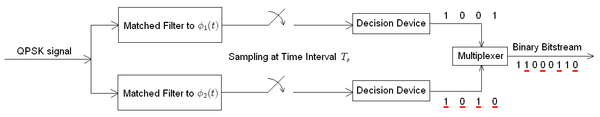Receiver structure for QPSK. The matched filters can be replaced with correlators. Each detection device uses a reference threshold value to determine whether a 1 or 0 is detected.

### Bit error rate

Although QPSK can be viewed as a quaternary modulation, it is easier to see it as two independently modulated quadrature carriers. With this interpretation, the even (or odd) bits are used to modulate the in-phase component of the carrier, while the odd (or even) bits are used to modulate the quadrature-phase component of the carrier. BPSK is used on both carriers and they can be independently demodulated.

As a result, the probability of bit-error for QPSK is the same as for BPSK:$P_b = Q\left(\sqrt{\frac{2E_b}{N_0}}\right).$

However, in order to achieve the same bit-error probability as BPSK, QPSK uses twice the power (since two bits are transmitted simultaneously).

The symbol error rate is given by:$\,\!P_s$$= 1 - \left( 1 - P_b \right)^2$$= 2Q\left( \sqrt{\frac{E_s}{N_0}} \right) - Q^2 \left( \sqrt{\frac{E_s}{N_0}} \right)^2$.

If the signal-to-noise ratio is high (as is necessary for practical QPSK systems) the probability of symbol error may be approximated:$P_s \approx 2 Q \left( \sqrt{\frac{E_s}{N_0}} \right )$

### QPSK signal in the time domain

The modulated signal is shown below for a short segment of a random binary data-stream. The two carrier waves are a cosine wave and a sine wave, as indicated by the signal-space analysis above. Here, the odd-numbered bits have been assigned to the in-phase component and the even-numbered bits to the quadrature component (taking the first bit as number 1). The total signal — the sum of the two components — is shown at the bottom. Jumps in phase can be seen as the PSK changes the phase on each component at the start of each bit-period. The topmost waveform alone matches the description given for BPSK above.Timing diagram for QPSK. The binary data stream is shown beneath the time axis. The two signal components with their bit assignments are shown the top and the total, combined signal at the bottom. Note the abrupt changes in phase at some of the bit-period boundaries.

The binary data that is conveyed by this waveform is: 1 1 0 0 0 1 1 0.

• The odd bits, highlighted here, contribute to the in-phase component: 1 1 0 0 0 1 1 0
• The even bits, highlighted here, contribute to the quadrature-phase component: 1 1 0 0 0 1 1 0

### Variants

#### Offset QPSK (OQPSK)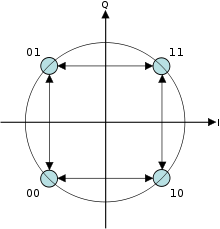Signal doesn't cross zero, because only one bit of the symbol is changed at a time

Offset quadrature phase-shift keying (OQPSK) is a variant of phase-shift keying modulation using 4 different values of the phase to transmit. It is sometimes called Staggered quadrature phase-shift keying (SQPSK).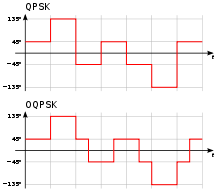Difference of the phase between QPSK and OQPSK

Taking four values of the phase (two bits) at a time to construct a QPSK symbol can allow the phase of the signal to jump by as much as 180° at a time. When the signal is low-pass filtered (as is typical in a transmitter), these phase-shifts result in large amplitude fluctuations, an undesirable quality in communication systems. By offsetting the timing of the odd and even bits by one bit-period, or half a symbol-period, the in-phase and quadrature components will never change at the same time. In the constellation diagram shown on the right, it can be seen that this will limit the phase-shift to no more than 90° at a time. This yields much lower amplitude fluctuations than non-offset QPSK and is sometimes preferred in practice.

The picture on the right shows the difference in the behavior of the phase between ordinary QPSK and OQPSK. It can be seen that in the first plot the phase can change by 180° at once, while in OQPSK the changes are never greater than 90°.

The modulated signal is shown below for a short segment of a random binary data-stream. Note the half symbol-period offset between the two component waves. The sudden phase-shifts occur about twice as often as for QPSK (since the signals no longer change together), but they are less severe. In other words, the magnitude of jumps is smaller in OQPSK when compared to QPSK.Timing diagram for offset-QPSK. The binary data stream is shown beneath the time axis. The two signal components with their bit assignments are shown the top and the total, combined signal at the bottom. Note the half-period offset between the two signal components.

#### π/4–QPSK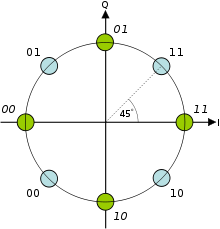Dual constellation diagram for π/4-QPSK. This shows the two separate constellations with identical Gray coding but rotated by 45° with respect to each other.

This variant of QPSK uses two identical constellations which are rotated by 45° (π / 4 radians, hence the name) with respect to one another. Usually, either the even or odd symbols are used to select points from one of the constellations and the other symbols select points from the other constellation. This also reduces the phase-shifts from a maximum of 180°, but only to a maximum of 135° and so the amplitude fluctuations of π / 4–QPSK are between OQPSK and non-offset QPSK.

One property this modulation scheme possesses is that if the modulated signal is represented in the complex domain, it does not have any paths through the origin. In other words, the signal does not pass through the origin. This lowers the dynamical range of fluctuations in the signal which is desirable when engineering communications signals.

On the other hand, π / 4–QPSK lends itself to easy demodulation and has been adopted for use in, for example, TDMA cellular telephone systems.

The modulated signal is shown below for a short segment of a random binary data-stream. The construction is the same as above for ordinary QPSK. Successive symbols are taken from the two constellations shown in the diagram. Thus, the first symbol (1 1) is taken from the 'blue' constellation and the second symbol (0 0) is taken from the 'green' constellation. Note that magnitudes of the two component waves change as they switch between constellations, but the total signal's magnitude remains constant. The phase-shifts are between those of the two previous timing-diagrams.Timing diagram for π/4-QPSK. The binary data stream is shown beneath the time axis. The two signal components with their bit assignments are shown the top and the total, combined signal at the bottom. Note that successive symbols are taken alternately from the two constellations, starting with the 'blue' one.

#### SOQPSK

The license-free shaped-offset QPSK (SOQPSK) is interoperable with Feher-patented QPSK (FQPSK), in the sense that an integrate-and-dump offset QPSK detector produces the same output no matter which kind of transmitter is used .

These modulations carefully shape the I and Q waveforms such that they change very smoothly, and the signal stays constant-amplitude even during signal transitions. (Rather than traveling instantly from one symbol to another, or even linearly, it travels smoothly around the constant-amplitude circle from one symbol to the next.)

The standard description of SOQPSK-TG involves ternary symbols.

#### DPQPSK

Dual-polarization quadrature phase shift keying (DPQPSK) or dual-polarization QPSK - involves the polarization multiplexing of two different QPSK signals, thus improving the spectral efficiency by a factor of 2. This is a cost-effective alternative, to utilizing 16-PSK instead of QPSK to double the spectral efficiency.

## Higher-order PSK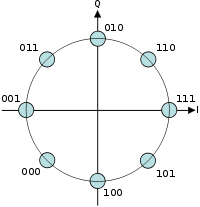Constellation diagram for 8-PSK with Gray coding.

Any number of phases may be used to construct a PSK constellation but 8-PSK is usually the highest order PSK constellation deployed. With more than 8 phases, the error-rate becomes too high and there are better, though more complex, modulations available such as quadrature amplitude modulation (QAM). Although any number of phases may be used, the fact that the constellation must usually deal with binary data means that the number of symbols is usually a power of 2 — this allows an equal number of bits-per-symbol.

### Bit error rate

For the general M-PSK there is no simple expression for the symbol-error probability if M > 4. Unfortunately, it can only be obtained from:$P_s = 1 - \int_{-\frac{\pi}{M}}^{\frac{\pi}{M}}p_{\theta_{r}}\left(\theta_{r}\right)d\theta_{r}$

where$p_{\theta_{r}}\left(\theta_r\right) = \frac{1}{2\pi}e^{-2\gamma_{s}\sin^{2}\theta_{r}}\int_{0}^{\infty}Ve^{-\left(V-\sqrt{4\gamma_{s}}\cos\theta_{r}\right)^{2}/2}dV$,$V = \sqrt{r_1^2 + r_2^2}$,$\theta_r = \tan^{-1}\left(r_2/r_1\right)$,$\gamma_{s} = \frac{E_{s}}{N_{0}}$ and$r_1 \sim{} N\left(\sqrt{E_s},N_{0}/2\right)$ and$r_2 \sim{} N\left(0,N_{0}/2\right)$ are jointly-Gaussian random variables.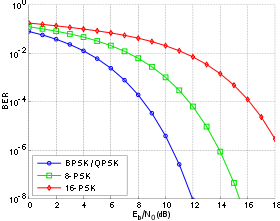Bit-error rate curves for BPSK, QPSK, 8-PSK and 16-PSK, AWGN channel.

This may be approximated for high M and high Eb / N0 by:$P_s \approx 2Q\left(\sqrt{2\gamma_s}\sin\frac{\pi}{M}\right)$.

The bit-error probability for M-PSK can only be determined exactly once the bit-mapping is known. However, when Gray coding is used, the most probable error from one symbol to the next produces only a single bit-error and$P_b \approx \frac{1}{k}P_s$.

(Using Gray coding allows us to approximate the Lee distance of the errors as the Hamming distance of the errors in the decoded bitstream, which is easier to implement in hardware.)

The graph on the left compares the bit-error rates of BPSK, QPSK (which are the same, as noted above), 8-PSK and 16-PSK. It is seen that higher-order modulations exhibit higher error-rates; in exchange however they deliver a higher raw data-rate.

Bounds on the error rates of various digital modulation schemes can be computed with application of the union bound to the signal constellation.

## Differential phase-shift keying (DPSK)

### Differential encoding

Differential phase shift keying (DPSK) is a common form of phase modulation that conveys data by changing the phase of the carrier wave. As mentioned for BPSK and QPSK there is an ambiguity of phase if the constellation is rotated by some effect in the communications channel through which the signal passes. This problem can be overcome by using the data to change rather than set the phase.

For example, in differentially-encoded BPSK a binary '1' may be transmitted by adding 180° to the current phase and a binary '0' by adding 0° to the current phase. Another variant of DPSK is Symmetric Differential Phase Shift keying, SDPSK, where encoding would be +90° for a '1' and -90° for a '0'.

In differentially-encoded QPSK (DQPSK), the phase-shifts are 0°, 90°, 180°, -90° corresponding to data '00', '01', '11', '10'. This kind of encoding may be demodulated in the same way as for non-differential PSK but the phase ambiguities can be ignored. Thus, each received symbol is demodulated to one of the M points in the constellation and a comparator then computes the difference in phase between this received signal and the preceding one. The difference encodes the data as described above. Symmetric Differential Quadrature Phase Shift Keying (SDQPSK) is like DQPSK, but encoding is symmetric, using phase shift values of -135°, -45°, +45° and +135°.

The modulated signal is shown below for both DBPSK and DQPSK as described above. In the figure, it is assumed that the signal starts with zero phase, and so there is a phase shift in both signals at t = 0.Timing diagram for DBPSK and DQPSK. The binary data stream is above the DBPSK signal. The individual bits of the DBPSK signal are grouped into pairs for the DQPSK signal, which only changes every Ts = 2Tb.

Analysis shows that differential encoding approximately doubles the error rate compared to ordinary M-PSK but this may be overcome by only a small increase in Eb / N0. Furthermore, this analysis (and the graphical results below) are based on a system in which the only corruption is additive white Gaussian noise(AWGN). However, there will also be a physical channel between the transmitter and receiver in the communication system. This channel will, in general, introduce an unknown phase-shift to the PSK signal; in these cases the differential schemes can yield a better error-rate than the ordinary schemes which rely on precise phase information.

### Demodulation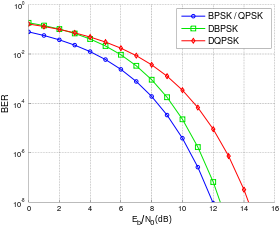BER comparison between DBPSK, DQPSK and their non-differential forms using gray-coding and operating in white noise.

For a signal that has been differentially encoded, there is an obvious alternative method of demodulation. Instead of demodulating as usual and ignoring carrier-phase ambiguity, the phase between two successive received symbols is compared and used to determine what the data must have been. When differential encoding is used in this manner, the scheme is known as differential phase-shift keying (DPSK). Note that this is subtly different to just differentially-encoded PSK since, upon reception, the received symbols are not decoded one-by-one to constellation points but are instead compared directly to one another.

Call the received symbol in the kth timeslot rk and let it have phase φk. Assume without loss of generality that the phase of the carrier wave is zero. Denote the AWGN term as nk. Then$r_k = \sqrt{E_s}e^{j\phi_k} + n_k$.

The decision variable for the k − 1th symbol and the kth symbol is the phase difference between rk and rk − 1. That is, if rk is projected onto rk − 1, the decision is taken on the phase of the resultant complex number:$r_kr_{k-1}^{*} = E_se^{j\left(\theta_k - \theta_{k-1}\right)} + \sqrt{E_s}e^{j\theta_k}n_{k-1}^{*} + \sqrt{E_s}e^{-j\theta_{k-1}}n_k + n_kn_{k-1}$

where superscript * denotes complex conjugation. In the absence of noise, the phase of this is θk − θk − 1, the phase-shift between the two received signals which can be used to determine the data transmitted.

The probability of error for DPSK is difficult to calculate in general, but, in the case of DBPSK it is:$P_b = \frac{1}{2}e^{-E_b/N_0},$

which, when numerically evaluated, is only slightly worse than ordinary BPSK, particularly at higher Eb / N0 values.

Using DPSK avoids the need for possibly complex carrier-recovery schemes to provide an accurate phase estimate and can be an attractive alternative to ordinary PSK.

In optical communications, the data can be modulated onto the phase of a laser in a differential way. The modulation is a laser which emits a continuous wave, and a Mach-Zehnder modulator which receives electrical binary data. For the case of BPSK for example, the laser transmits the field unchanged for binary '1', and with reverse polarity for '0'. The demodulator consists of a delay line interferometer which delays one bit, so two bits can be compared at one time. In further processing, a photo diode is used to transform the optical field into an electric current, so the information is changed back into its original state.

The bit-error rates of DBPSK and DQPSK are compared to their non-differential counterparts in the graph to the right. The loss for using DBPSK is small enough compared to the complexity reduction that it is often used in communications systems that would otherwise use BPSK. For DQPSK though, the loss in performance compared to ordinary QPSK is larger and the system designer must balance this against the reduction in complexity.

### Example: Differentially-encoded BPSK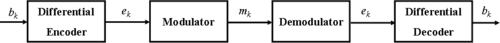Differential encoding/decoding system diagram.

At the kth time-slot call the bit to be modulated bk, the differentially-encoded bit ek and the resulting modulated signal mk(t). Assume that the constellation diagram positions the symbols at ±1 (which is BPSK). The differential encoder produces:$\,e_k = e_{k-1}\oplus{}b_k$

where$\oplus{}$ indicates binary or modulo-2 addition.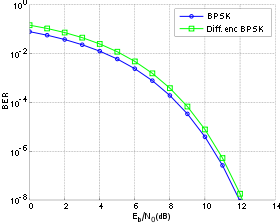BER comparison between BPSK and differentially-encoded BPSK with gray-coding operating in white noise.

So ek only changes state (from binary '0' to binary '1' or from binary '1' to binary '0') if bk is a binary '1'. Otherwise it remains in its previous state. This is the description of differentially-encoded BPSK given above.

The received signal is demodulated to yield ek = ±1 and then the differential decoder reverses the encoding procedure and produces:$\,b_k = e_{k}\oplus{}e_{k-1}$ since binary subtraction is the same as binary addition.

Therefore, bk = 1 if ek and ek − 1 differ and bk = 0 if they are the same. Hence, if both ek and ek − 1 are inverted, bk will still be decoded correctly. Thus, the 180° phase ambiguity does not matter.

Differential schemes for other PSK modulations may be devised along similar lines. The waveforms for DPSK are the same as for differentially-encoded PSK given above since the only change between the two schemes is at the receiver.

The BER curve for this example is compared to ordinary BPSK on the right. As mentioned above, whilst the error-rate is approximately doubled, the increase needed in Eb / N0 to overcome this is small. The increase in Eb / N0 required to overcome differential modulation in coded systems, however, is larger - typically about 3 dB. The performance degradation is a result of noncoherent transmission - in this case it refers to the fact that tracking of the phase is completely ignored.

## Channel capacity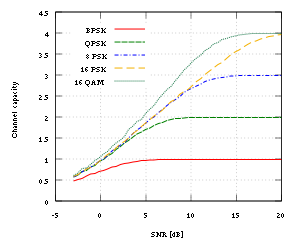Given a fixed bandwidth, channel capacity vs. SNR for some common modulation schemes

Like all M-ary modulation schemes with M = 2b symbols, when given exclusive access to a fixed bandwidth, the channel capacity of any phase shift keying modulation scheme rises to a maximum of b bits per symbol as the signal-to-noise ratio increases.

Wikimedia Foundation. 2010.

Нужна курсовая?

### Look at other dictionaries:

• Phase shift keying — Traduction terminée Phase shift keying → …   Wikipédia en Français

• Phase Shift Keying —   [engl.], Phasenverschiebung …   Universal-Lexikon

• Phase Shift Keying — Die rote Sinusschwingung ist gegenüber der blauen Sinusschwingung um ein Viertel der Periodendauer verzögert. Im komplexen zweiseitigen Frequenzspektrum zeigt sich das als eine 90° Drehung der beiden Spektrallinien, die den Träger darstellen. Die …   Deutsch Wikipedia

• Phase-shift keying — Le Phase shift keying (ou PSK, soit « modulation par déplacement de phase ») désigne une famille de formes de modulations numériques qui ont toutes pour principe de véhiculer de l information binaire via la phase d un signal de… …   Wikipédia en Français

• phase-shift keying — fazės manipuliavimas statusas T sritis automatika atitikmenys: angl. phase shift keying vok. Phasentastung, f rus. фазовая манипуляция, f pranc. modulation par déplacement de phase, f …   Automatikos terminų žodynas

• Binary-phase shift keying — Phase shift keying Traduction terminée Phase shift keying → …   Wikipédia en Français

• Binary phase-shift keying — Phase shift keying Traduction terminée Phase shift keying → …   Wikipédia en Français

• Differential phase-shift keying — Phase shift keying Traduction terminée Phase shift keying → …   Wikipédia en Français

• Quad phase shift keying — Phase shift keying Traduction terminée Phase shift keying → …   Wikipédia en Français

• Quadrature phase-shift keying — Phase shift keying Traduction terminée Phase shift keying → …   Wikipédia en Français